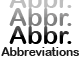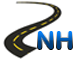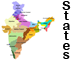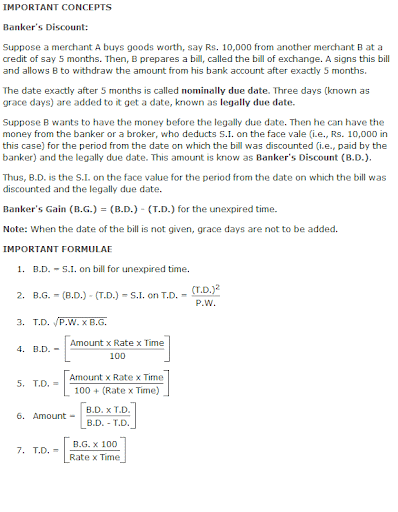Banker's Algorithm -List of AbbreviationsNational Highways in IndiaStates & Union Territories of IndiaCurrency of All Countries

# Banker’s Algorithm

Overview:Sample Questions:

1.If the true discount on a certain sum due 6 months hence at 15% is Rs 120.What is the bankers discount on the same for same time and the same rate.
A. 120 B. 130
C. 129 D. 126

Ans: C

2.The bankers discount on Rs 1800 at 12 % per annum is equal to the true discount on Rs 1872 for the same time at the same rate .Find the time.
A. 4 Months B. 3 Months
C. 9 Months D. 6 Months

Ans: A

3.The bankers discount and true discount on a sum of money due 8 months hence are Rs.120 & Rs.110 resp. Find the sum & the rate per cent
A. 132% B. 137%
C. 129% D. 137/11%

Ans: D

4.The Bankers discount on Rs 1650 due a certain time hence is Rs 165. find the true discount and the bankers gain.
A. Rs.12 B. Rs.13
C. Rs.15 D. Rs.16

Ans: C

5.The Present worth of a bill due something hence is Rs 1110 and the true discount on the bill is Rs.110 . Find the bankes discount & the bankers gain.
A. Rs.121 B. Rs.125
C. Rs.134 D. Rs.126

Ans: A

6.What rate percent does a man get for his money when in discounting
A. 132% B. 131/5%
C. 131/3% D. 137/11%

Ans: C

7.A bill for Rs.6000 is drawn on July 14 at 5 months . It is discounted on 5th October at 10%.Find the bankers discount true discount, bankers gain and the money that the holder of the bill receives.
A. 5890 B. 5880
C. 8550 D. 6885

Ans: B

2. The bankers gain on a certain sum due 1 ½ year hence is 3/25 of the bankers discount .The rate percent is:
A. 91/9% B. 89/5%
C. 76/3% D. 37/11%

Ans: A

3. The bankers gain of a certain sum due 2 years hence at 10% per annum is Rs 24 .The percent worth is:
A. 450 B. 600
C. 550 D. 650

Ans: BClick Here to Find Latest Jobs and Current Affairs

Updated: May 31, 2013 — 10:48 am